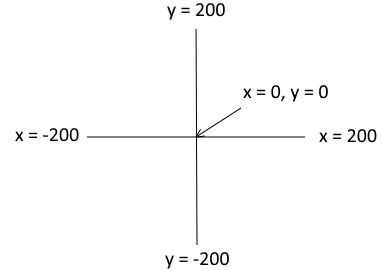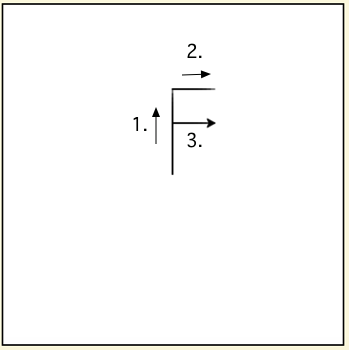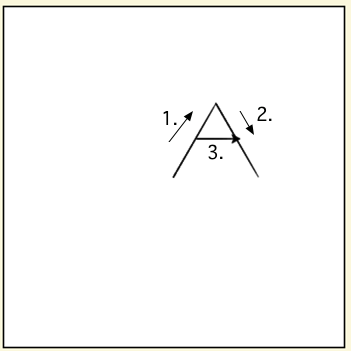# 4.6. Turtle Coordinates¶

The space that the turtle draws in is 400 by 400 pixels. The center of the space is at x=0, y=0.The coordinates for the drawing space. Note that the center is x = 0 and y = 0.¶

The program below draws a snowman on a blue background by drawing 4 circles. It positions each of the circles using goto(x,y) to move the turtle to exactly where we want it centered. To draw a circle, we use a trick: we use pensize to make a really big (circular) pen, then do forward(0) to make a dot of the current color.

The program below uses the goto(x,y) to move to the top left corner before drawing a square that nearly fills the drawing space. It uses fillcolor, begin_fill, and end_fill to fill in the shape as it draws it.

Mixed up programs

The following program uses a turtle to draw a capital F as shown below, but the lines are mixed up. The program should do all necessary set-up: import the turtle module, get the space to draw on, and create the turtle. It should draw the lines in the order shown by the numbers in the picture on the left.

Drag the needed blocks of statements from the left column to the right column and put them in the right order. There may be extra blocks that are not needed in a correct solution. Then click on Check to see if you are right.The following program uses a turtle to draw a capital A as shown below, but the lines are mixed up. The program should do all necessary set-up: import the turtle module, get the space to draw on, and create the turtle. It should draw the lines in the order shown by the numbers in the picture on the left. Drag the needed blocks of statements from the left column to the right column and put them in the right order. There may be additional blocks that are not needed in a correct solution. Then click on Check to see if you are right.